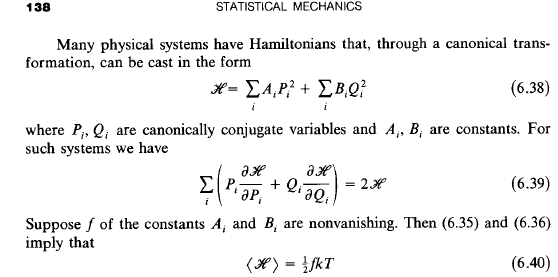# Validity of Equipartition Theorem

So I have this question that goes like this, for a classical 1D system we are given an Hamiltonian of the form of an Harmonic Oscilator. However the term for the potential is infite when ##x\leq0## and the usual harmonical oscillator potential otherwise. The question is: is the equipartition theorem valid for each term of the Hamiltonian?

Attempt at a solution
Now for all I've read the only condition on the equipartition theorem is that the system must be in thermal equilibrium, so I see no reason for it not to be valid, although I don't know how to properly justify this. However, I have a gut feeling that the infinite potential will make the theorem invalid, but once again I have no idea how to justify this.

TSny
Homework Helper
Gold Member
It will help if you give some information about the type and level of the course associated with this problem. What specific concepts have you covered that you believe would be relevant to this question?

Undergrad Statistical Physics course, we covered the demonstration of the equipartition theorem, if that's of any help.

TSny
Homework Helper
Gold Member
Undergrad Statistical Physics course, we covered the demonstration of the equipartition theorem, if that's of any help.
What mathematical concepts did you use to demonstrate the theorem? Can you use the same concepts for this example?

Our syllabus follows Statistical Mechanics (Kerson, Huang) so that's the demonstration we used. As for the mathematical concepts used, integrals and averages I guess?

For the sake of brevity here's a printscreen of the last part of the demonstration.The Hamiltonian we are given is of the same form as shown in (6.38) where A is ##1/2m## and B is infinite for ##z\leq0## and ##\alpha/2## otherwise. The two equations referenced, (6.35) and (6.36) simply state that the average of each term of (6.39) is equal to ##kT##. However I am pretty sure that (6.36) will not be equal to ##kT## when B is infinite.

TSny
Homework Helper
Gold Member
Have you tried explicitly calculating ##\left<BQ^2 \right> ## for your problem?

I'll give it a shot, the problem is that in my textbook they don't specify the Hamiltonian they use but I'll try doing the calculations myself. Do you mind giving me the final answer so I have something to compare my calculations to when I'm done?

TSny
Homework Helper
Gold Member
I'm reluctant to give you my answer since it's your problem. (I could be wrong, anyway.) But show us your calculation when you're done and we can check it.

I just have one question regarding the calculations. The integrals are defined for ##H<E## or we can also use ##E<H<E+\Delta##. In a region where a term of ##H## is infite, isn't the entire integral "broken" right off the bat?

I mean, unless there's something I'm missing, ##dH/dQ_i## comes out as infinite and so the integral would also be infinite for ##x\leq0##. In fact, these kinds of potentials were used throughout my course as confining potentials, so it would make no physical sense for the equipartition theorem to be valid on that range of ##x## since that infinity is there to confine the physical system to ##x>0## in the first place.

Last edited:
TSny
Homework Helper
Gold Member
The infinite potential for ##x < 0## just means that only states with non-negative ##x## are allowed. So, the allowable states in phase space all have non-negative ##x##. Thus, integrations over phase space are restricted to integrating ##x## from 0 to infinity.

Why not use the canonical ensemble (distribution) to directly evaluate ##\left< T \right>## and ##\left< U \right>##, where ##T## is the kinetic energy part of the Hamiltonian and ##U## is the potential energy part of the Hamiltonian?

•LizardWizard
Thanks, I'll do that, but I think the trick here was evaluating the consequences of the infinite potential. With positive x it just becomes an harmonic oscillator and I think simple conservation of energy implies the equipartition theorem. I didn't already do the calculations because my professor usually tends to asks us more to discuss the physical implications of a system rather than just mathematical demonstrations so I'm taking that approach for the most part. I'll do the calculations anyway but from now on it looks like a straightforward demonstration. Thank you for the help.